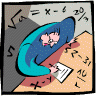## Mathematics, Department of## Dissertations, Theses, and Student Research Papers in Mathematics

Solomon Akesseh

Brian Harbourne

8-2017

#### Comments

A DISSERTATION Presented to the Faculty of The Graduate College at the University of Nebraska In Partial Fulfillment of Requirements For the Degree of Doctor of Philosophy, Major: Mathematics, Under the Supervision of Professor Brian Harbourne. Lincoln, Nebraska: August, 2017

Copyright (c) 2017 Solomon Akesseh

#### Abstract

Fat points and their ideals have stimulated a lot of research but this dissertation concerns itself with aspects of only two of them, broadly categorized here as, the ideal containments and polynomial interpolation problems.

Ein-Lazarsfeld-Smith and Hochster-Huneke cumulatively showed that for all ideals I in k[Pn], I(mn) ⊆ Im for all m ∈ N. Over the projective plane, we obtain I(4)< ⊆ I2. Huneke asked whether it was the case that I(3) ⊆ I2. Dumnicki, Szemberg and Tutaj-Gasinska show that if I is the saturated homogeneous radical ideal of the 12 points of the Hesse configuration, then I(3) ̸⊆ I2. Since then, additional examples have been found, but all of them, are the intersection loci of lines. Here we extend all the examples of I(3) ̸⊆ I2 to points that are not directly the intersection loci of lines but are the intersection loci of curves.

In the case of the interpolation problem, this dissertation makes the following contribution. Let k be an algebraically closed field of arbitrary characteristic. Let q1,...,qr be a set of not necessarily general points and let p1,...,ps be a set of general points in P2, r+s ≤ 8. Let X be a blow up of the points with e1,...,er and E1, . . . , Es the corresponding exceptional curves. Write e = a1e1 + · · · + arer and E = b1E1 +···+bsEs. For the two linear systems [dL−e−E] and [dL−e] with [dL − e − E] ⊆ [dL − e], we give a condition sufficient to guarantee that

h0(X, dL − e − E) > max{0, h0(X, dL − e) − ∑((bi+1) choose 2)} and another condition necessary for h0(X, dL − e − E) > max{0, h0(X, dL − e) − ∑((bi+1) choose 2)}. When r =7,s=1,d=3,aj =1,1≤j≤7 and b=2, we connect the discussion to quasi-elliptic fibrations and show that when q1 + · · · + q7 is reduced, then h0(X,3L−e1 −···−e7 −2E) > max{0,h0(X,3L−e1 −···−e7)−3} if and only if q1 + · · · + q7 is the union of the seven points of the Fano plane. Allowing infinitely near points, we obtain nonreduced subschemes q1 + · · · + q7, consisting of essentially distinct points, that form part of the base loci of quasi-elliptic fibrations such that h0(X,3L−e1 −···−e7 −2E) > max{0,h0(X,3L−e1 −···−e7)−3}.

Adviser: Brian Harbourne

COinS